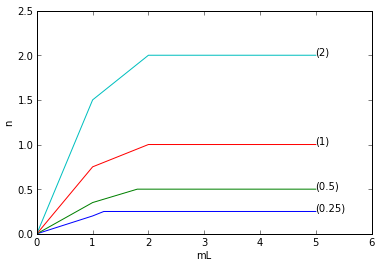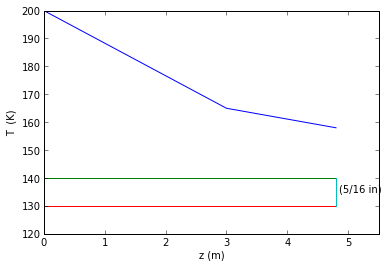# chapter 2 :Steady state Conduction in one dimension¶

### Example 2.1 Page No.42¶

In :
T3=-10.00		# temperature of inside wall in degree Fahrenheit
T0=70.0 	  	# temperature of outside wall in degree Fahrenheit
dT=T0-T3          # overall temperature difference
k1=0.38           # brick masonry
k2=0.02           # glass fibre
k3=0.063          # plywood
dx1=4/12.0          # thickness of brick layer in ft
dx2=3.5/12.0        # thickness of glass fibre layer in ft
dx3=0.5/12.0        # thickness of plywood layer in ft
A=1.0 # cross sectional area taken as 1 ft**2

R1=dx1/(k1*A) # resistance of brick layer in (hr.degree Rankine)/BTU
R2=dx2/(k2*A) # resistance of glass fibre layer in (hr.degree Rankine)/BTU
R3=dx3/(k3*A) # resistance of plywood layer in (hr.degree Rankine)/BTU
qx=(T0-T3)/(R1+R2+R3)

print"Resistance of brick layer is  ",round(R1,3),"(hr.degree Rankine)/BTU"
print"Resistance of glass fibre layer is ",round(R2,1),"(hr.degree Rankine)/BTU"
print"Resistance of plywood layer is  ",round(R3,3),"(hr.degree Rankine)/BTU"
print"Heat transfer through the composite wall is ",round(qx,2),"(hr.degree Rankine)/BTU"

Resistance of brick layer is   0.877 (hr.degree Rankine)/BTU
Resistance of glass fibre layer is  14.6 (hr.degree Rankine)/BTU
Resistance of plywood layer is   0.661 (hr.degree Rankine)/BTU
Heat transfer through the composite wall is  4.96 (hr.degree Rankine)/BTU


### Example 2.2 Page No.45¶

In :
k1=0.45           # thermal conductivity of brick
k2a=0.15          # thermal conductivity of pine
k3=0.814         # thermal conductivity of plaster board
k2b=0.025       # thermal conductivity of air from appendix table D1
A1=0.41*3       # cross sectional area of brick layer
A2a=0.038*3     # cross sectional area of wall stud
A2b=(41-3.8)*0.01*3 # cross sectional area of air layer
A3=0.41*3     # cross sectional area of plastic layer
dx1=0.1        # thickness of brick layer in m
dx2=0.089      # thickness of wall stud and air layer in m
dx3=0.013      # thickness of plastic layer in m

R1=dx1/(k1*A1) # Resistance of brick layer in K/W
R2=dx2/(k2a*A2a+k2b*A2b) # Resistance of wall stud and air layer in K/W
R3=dx3/(k3*A3) # Resistance of plastic layer in K/W
T1=25          # temperature of inside wall in degree celsius
T0=0           # temperature of outside wall in degree celsius
qx=(T1-T0)/(R1+R2+R3) # heat transfer through the composite wall in W

print"Resistance of brick layer is ",round(R1,3),"k/W"
print"Resistance of wall stud and air layer is  ",round(R2,2),"k/W"
print"Resistance of plastic layer is ",round(R3,3),"k/W"
print"Heat transfer through the composite wall is",round(qx,1),"W"

Resistance of brick layer is  0.181 k/W
Resistance of wall stud and air layer is   1.98 k/W
Resistance of plastic layer is  0.013 k/W
Heat transfer through the composite wall is 11.5 W


### Example 2.3 Page No. 50¶

In :
k1=24.8      # thermal conductivity of 1C steel in BTU/(hr.ft.degree Rankine)from appendix table B2
k2=0.02      # thermal conductivity of styrofoam steel in BTU/(hr.ft.degree Rankine)
k3=0.09      # thermal conductivity of fibreglass in BTU/(hr.ft.degree Rankine)
hc1=0.79     # convection coefficient between the air and the vertical steel wall in BTU/(hr.ft**2.degree Rankine)
hc2=150.0      # the convection coefficient between the ice water and the fiberglass
A=1.0          # calculation based on per square foot
dx1=0.04/12.0  #  thickness of steel in ft
dx2=0.75/12.0  # thickness of styrofoam in ft
dx3=0.25/12.0  # thickness of fiberglass in ft

Rc1=1/(hc1*A) # Resistance from air to sheet metal
Rk1=dx1/(k1*A) # Resistance of steel layer
Rk2=dx2/(k2*A) # Resistance of styrofoam layer
Rk3=dx3/(k3*A) # Resistance of fiberglass layer
Rc2=1/(hc2*A) # Resistance from ice water to fiberglass
U=1/(Rc1+Rk1+Rk2+Rk3+Rc2) # overall heat transfer coefficient
T_inf1=90     # temperature of air in degree F
T_inf2=32    # temperature of mixture of ice and water in degree F
q=U*A*(T_inf1-T_inf2)

print"Resistance from air to sheet metal:  ",round(Rc1,3),"degree F.hr/BTU"
print"Resistance of steel layer is ",round(Rk1,4),"degree F.hr/BTU"
print"Resistance of styrofoam layer is ",round(Rk2,3),"degree F.hr/BTU"
print"Resistance of fiberglass layer is ",round(Rk3,3),"degree F.hr/BTU"
print"Resistance from ice water to fiberglass is ",round(Rc2,4),"degree F.hr/BTU"
print"The overall heat transfer coefficient is  ",round(U,3),"BTU/hr ft**2"
print"The heat transfer rate is %.1f BTU/hr",round(q,2),"BTU/hr"

Resistance from air to sheet metal:   1.266 degree F.hr/BTU
Resistance of steel layer is  0.0001 degree F.hr/BTU
Resistance of styrofoam layer is  3.125 degree F.hr/BTU
Resistance of fiberglass layer is  0.231 degree F.hr/BTU
Resistance from ice water to fiberglass is  0.0067 degree F.hr/BTU
The overall heat transfer coefficient is   0.216 BTU/hr ft**2
The heat transfer rate is %.1f BTU/hr 12.53 BTU/hr


### Example 2.4 Page No 55.¶

In :
k=14.4       # thermal conductivity of 304 stainless steel in W/(m.K) from appendix table B2
D2=32.39     #Diameter (cm)
D1=29.53
T1=40         #Temprature
T2=38

import math
Qr_per_length=(2*3.14*k)*(T1-T2)/math.log(D2/D1)#format(6)

print"The heat transfer through the pipe wall per unit length of pipe is ",round(Qr_per_length/1000,2),"kw/m"

The heat transfer through the pipe wall per unit length of pipe is  1.96 kw/m


### Example 2.5 Page NO. 58¶

In :
k1=231             # thermal conductivity of copper in BTU/(hr.ft.degree Rankine)from appendix table B1
k2=0.02            # thermal conductivity of insuLtion in BTU/(hr.ft.degree Rankine)
D2=1.125/12       # outer diameter in ft
D1=0.08792        # inner diameter in ft
t=0.5/12          # wall thickness of insulation in ft
R3=R2+t
LRk1=(log(R2/R1))/(2*3.14*k1) # product of length and copper layer resistance
LRk2=(log(R3/R2))/(2*3.14*k2) # product of length and insulation layer resistance
T1=40 # temperature of inside wall of tubing in degree fahrenheit
T3=70 # temperature of surface temperature of insulation degree fahrenheit
q_per_L=(T1-T3)/(LRk1+LRk2) # heat transferred per unit length in BTU/(hr.ft)

print"The heat transferred per unit length is ",round(q_per_L,2)," BTU/(hr.ft"

The heat transferred per unit length is  -5.92  BTU/(hr.ft


### Example 2.6 Page No 63¶

In :
k12=24.8      # thermal conductivity of 1C steel in BTU/(hr.ft.degree Rankine)from appendix table B2
k23=.023      # thermal conductivity of glass wool insulation in BTU/(hr.ft.degree Rankine)from appendix table B3
D2=6.625/12.0  # outer diameter in ft
D1=0.5054    # inner diameter in ft
t=2/12.0        # wall thickness of insulation in ft
D3=D2+t
hc1=12        # convection coefficient between the air and the pipe wall in BTU/(hr. sq.ft.degree Rankine).
hc2=1.5       # convection coefficient between the glass wool and the ambient air in  BTU/(hr. sq.ft.degree Rankine).

U=1/((1/hc1)+(D1*log(D2/D1)/k12)+(D1*log(D3/D2)/k23)+(D1/(hc2*D3)))

print"Overall heat transfer coefficient is  ",round(U,4)," BTU/(hr.sq.ft. Fahrenheit)"

Overall heat transfer coefficient is   0.1596  BTU/(hr.sq.ft. Fahrenheit)


### Example 2.7 Page NO.72¶

In :
k=14.4       # thermal conductivity of 304 stainless steel in  W/(m.K)from appendix table B2
T1=543.0     # temperature in K at point 1
T2=460.0     # temperature in K at point 2
dT=T1-T2     # temperature difference between point 1 and 2
dz12=0.035   # distance between thermocouple 1 and 2 in cm

dz56=4.45    # distance between thermocouple 5 and 6 in cm
dz6i=3.81    # distance between thermocouple 6 and interface in cm
dz5i=dz56+dz6i # distance between thermocouple 5 and interface in cm
T5=374       # temperature in K at point 5
T6=366       # temperature in K at point 6
dzi7=2.45       # distance between thermocouple 7 and interface in cm
dz78=4.45       # distance between thermocouple 7 and 8 in cm
dzi8=dzi7+dz78  # distance between thermocouple 8 and interface in cm
T7=349          #  temperature in K at point 7
T8=337          # temperature in K at point 8

qz_per_A=k*dT/dz12 # heat flow calculated in W/m**2 calculated using Fourier's law
T_ial=T5-(dz5i*(T5-T6)/dz56) # temperature of aluminium interface in K
T_img=dzi8*(T7-T8)/dz78+T8   # temperature of magnesium interface in K
T_img_=355.8                 #Approx value in the book
Rtc=(T_ial-T_img_)/(qz_per_A)

print"The required thermal contact resistance is",round(Rtc,7),"K sq.m/W"

The required thermal contact resistance is 9.81e-05 K sq.m/W


### Example 2.8 Page No. 85¶

In [ ]:
%matplotlib inline

In :
import math
k=24.8                  # thermal conductivity of 1C steel in BTU/(hr.ft.degree Rankine)from appendix table B2
D=(5.0/16.0)/12.0           # diameter of the rod in ft
P=(math.pi*D)           # Circumference of the rod in ft
A=(math.pi/4)*D**2      # Cross sectional area of the rod in sq.ft
hc=1.0 # assuming the convective heat transfer coefficient as 1 BTU/(hr. sq.ft. degree Rankine)

m=math.sqrt(hc*P/(k*A))
L=(9/2.0)/12.0 # length of rod in ft
T_inf=70.0
T_w=200.0
dT=T_w-T_inf
const=dT/math.cosh(m*L)

qz=k*A*m*dT*tanh(m*L)

mL=m*L
efficiency=0.78       # from fig. 2.30

effectiveness=math.sqrt(k*P/(hc*A))*tanh(mL)

print"(b)The heat transferred is ",round(qz,2),"BTU/hr"
print"(c)The efficiency found from the graph in figure 2.30 is",efficiency
print"(d)The effectiveness is found to be",round(effectiveness,1)

import matplotlib.pyplot as plt
fig = plt.figure()

mL1=[0,1,1.2,5]
n1=[0,0.2,0.25,0.25]

mL2=[0,1,1.8,5]
n2=[0,0.35,0.5,0.5]

mL3=[0,1,2,5]
n3=[0,0.75,1,1]
mL4=[0,1,2,5]
n4=[0,1.5,2,2]

xlabel("mL")
ylabel("n  ")
plt.xlim((0,6))
plt.ylim((0,2.5))

ax.annotate('(0.25)', xy=(5,0.25))
ax.annotate('(0.5)', xy=(5,0.5))
ax.annotate('(1)', xy=(5,1))
ax.annotate('(2)', xy=(5,2))
a1=plot(mL1,n1)
a2=plot(mL2,n2)
a3=plot(mL3,n3)
a4=plot(mL4,n4)

import matplotlib.pyplot as plt
fig = plt.figure()

z1=[0,3,4.8]
T1=[200,165,158]
z2=[0,4.8]
T2=[140,140]
z3=[0,4.8]
T3=[130,130]
z4=[4.8,4.8]
T4=[140,130]

xlabel("z (m)")
ylabel("T  (K)")
plt.xlim((0,5.5))
plt.ylim((120,200))

ax.annotate('(5/16 in)', xy=(4.85,135))
a1=plot(z1,T1)
a2=plot(z2,T2)
a3=plot(z3,T3)
a4=plot(z4,T4)

(b)The heat transferred is  3.13 BTU/hr
(c)The efficiency found from the graph in figure 2.30 is 0.78
(d)The effectiveness is found to be 45.2### Example 2.9 Page No. 90¶

In :
k=136.0             # thermal conductivity of aluminium in BTU/(hr.ft.degree Rankine)from appendix table B1
L=9/(8*12.0)        #length in ft
W=9/(4*12.0)          #width in ft
delta=0.002604       #ft
hc=0.8             # the convective heat transfer coefficient estimated as 1 BTU/(hr.ft**2. degree Rankine)
T_w=1000.0             # the root temperature in degree fahrenheit
T_inf=90.0             # the ambient temperature in degree fahrenheit

import math
m=math.sqrt(hc/(k*delta))
P=2*W
A=2*delta*W
qz1=math.sqrt(hc*P*k*A)*(T_w-T_inf)*(sinh(m*L)+(hc/(m*k)*cosh(m*L)))/(cosh(m*L)+(hc/(m*k)*sinh(m*L)))
qz2=math.sqrt(k*A*hc*P)*(T_w-T_inf)*math.tanh(m*L)
Lc=L+delta
qz3=k*A*m*(T_w-T_inf)*math.tanh(m*L*(1+delta/Lc))

print"(a)The heat transferred is  ",round(qz1,2),"BTU/hr"
print"(b)The heat transferred is ",round(qz2,2),"BTU/hr      In the book the answer is incorrect"
print"(c)The heat transferred is ",round(qz3,2)," BTU/hr"

(a)The heat transferred is   26.12 BTU/hr
(b)The heat transferred is  25.43 BTU/hr      In the book the answer is incorrect
(c)The heat transferred is  26.1  BTU/hr


### Example 2.10 Page No 94¶

In :
k=8.32           # thermal conductivity  BTU/(hr.ft.degree Rankine)
hc=400.0           # the convective heat transfer coefficient given in BTU/(hr.ft**2. degree Rankine)

import math
delta_opt=0.55/(12*2)
Lc=math.sqrt(delta_opt*k/(0.583*hc))

A=Lc*delta_opt
parameter=Lc**1.5*math.sqrt(hc/(k*A))
efficiency=0.6
W=1/(2.0*12.0)   # width in ft
T_w=190.0       # wall temperature in degree fahrenheit
T_inf=58.0      # ambient temperature in degree fahrenheit
L=1.0           # length in ft
delta=W/2.0
q_ac=efficiency*hc*2*W*math.sqrt(L**2+delta**2)*(T_w-T_inf)

print"(a)The optimum length is  ",round(Lc*12,2),"inch"
print"(b)The actual heat transferred is ",round(q_ac,2),"BTU/hr.  NOTE: In the book answer is incorrect"

(a)The optimum length is   0.34 inch
(b)The actual heat transferred is  2640.57 BTU/hr.  NOTE: In the book answer is incorrect


### Example 2.11 Page No 95¶

In :
N=9            # number of fins
delta=0.003/2.0
L=0.025
Lc=L+delta
R=0.219/2
R2c=R+delta
R1=R-L
T_w=260       # root wall temperature in degree celsius
T_inf=27      # ambient temperature in degree celsius
hc=15
k=52          # thermal conductivity of cast iron in W/(m.K)from appendix table B2

import math
Ap=2*delta*Lc
As=2*math.pi*(R2c**2-R1**2)
radius_ratio=R2c/R1    # for finding efficiency from figure 2.38
variable=Lc**1.5*math.sqrt(hc/(k*Ap))
efficiency=0.93        # efficiency from figure 2.38
qf=N*efficiency*As*hc*(T_w-T_inf)
Sp=0.0127              # fin spacing
Asw=2*math.pi*R1*Sp*N     # exposed surface area
qw=hc*Asw*(T_w-T_inf)
q=qf+qw

H=N*(Sp+2*delta)
Aso=2*math.pi*R1*H       # surface area without fins
qo=hc*Aso*(T_w-T_inf)

effectiveness=q/qo     # effectiveness defined as ratio of heat transferred with fins to heat transferred without fins

print"(a)The total heat transferred from the cylinder is  ",round(q,0),"W"
print"(b)The Heat transferred without fins is W",round(qo,0),"W"
print"(c)The fin effectiveness is ",round(effectiveness,2)

(a)The total heat transferred from the cylinder is   1164.0 W
(b)The Heat transferred without fins is W 262.0 W
(c)The fin effectiveness is  4.44

In [ ]: